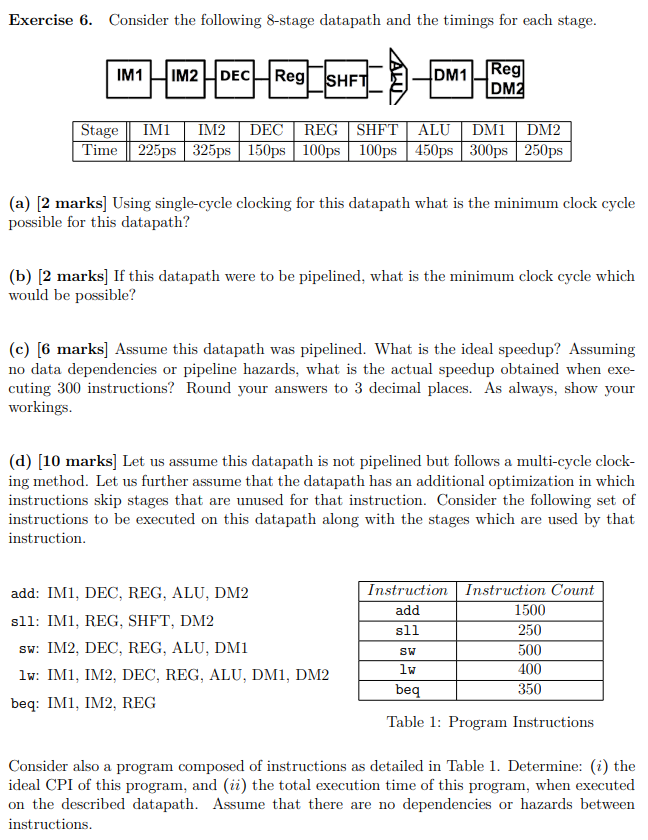# Question Exercise 6. Consider the following 8-stage datapath and the timings for each stage. IM1 IM2 DECH Reg SHFTI 一台 DM1_Reg DME Stage IM1 IM2 DEC REG SHFT ALU DM1 DM2 Time 225ps 325ps 150ps 100ps 100ps 450ps 300ps 250ps (a) [2 marks] Using single-cycle clocking for this datapath what is the minimum clock cycle possible for this datapath? (b) [2 marks] If this datapath were to be pipelined, what is the minimum clock cycle which would be possible? (c) [6 marks] Assume this datapath was pipelined. What is the ideal speedup? Assuming no data dependencies or pipeline hazards, what is the actual speedup obtained when exe- cuting 300 instructions? Round your answers to 3 decimal places. As always, show your workings. (d) (10 marks] Let us assume this datapath is not pipelined but follows a multi-cycle clock- ing method. Let us further assume that the datapath has an additional optimization in which instructions skip stages that are unused for that instruction. Consider the following set of instructions to be executed on this datapath along with the stages which are used by that instruction add: IM1, DEC, REG, ALU, DM2 sll: IM1, REG, SHFT, DM2 Sw: IM2, DEC, REG, ALU, DM1 lw: IMI, IM2, DEC, REG, ALU, DMI, DM2 beq: IMI, IM2, REG Instruction Instruction Count add 1500 sll 250 500 lw 400 beq 350 SW Table 1: Program Instructions Consider also a program composed of instructions as detailed in Table 1. Determine: (i) the ideal CPI of this program, and (ii) the total execution time of this program, when executed on the described datapath. Assume that there are no dependencies or hazards between instructions.4QPJWD The Asker · Computer ScienceTranscribed Image Text: Exercise 6. Consider the following 8-stage datapath and the timings for each stage. IM1 IM2 DECH Reg SHFTI 一台 DM1_Reg DME Stage IM1 IM2 DEC REG SHFT ALU DM1 DM2 Time 225ps 325ps 150ps 100ps 100ps 450ps 300ps 250ps (a) [2 marks] Using single-cycle clocking for this datapath what is the minimum clock cycle possible for this datapath? (b) [2 marks] If this datapath were to be pipelined, what is the minimum clock cycle which would be possible? (c) [6 marks] Assume this datapath was pipelined. What is the ideal speedup? Assuming no data dependencies or pipeline hazards, what is the actual speedup obtained when exe- cuting 300 instructions? Round your answers to 3 decimal places. As always, show your workings. (d) (10 marks] Let us assume this datapath is not pipelined but follows a multi-cycle clock- ing method. Let us further assume that the datapath has an additional optimization in which instructions skip stages that are unused for that instruction. Consider the following set of instructions to be executed on this datapath along with the stages which are used by that instruction add: IM1, DEC, REG, ALU, DM2 sll: IM1, REG, SHFT, DM2 Sw: IM2, DEC, REG, ALU, DM1 lw: IMI, IM2, DEC, REG, ALU, DMI, DM2 beq: IMI, IM2, REG Instruction Instruction Count add 1500 sll 250 500 lw 400 beq 350 SW Table 1: Program Instructions Consider also a program composed of instructions as detailed in Table 1. Determine: (i) the ideal CPI of this program, and (ii) the total execution time of this program, when executed on the described datapath. Assume that there are no dependencies or hazards between instructions.
More
Transcribed Image Text: Exercise 6. Consider the following 8-stage datapath and the timings for each stage. IM1 IM2 DECH Reg SHFTI 一台 DM1_Reg DME Stage IM1 IM2 DEC REG SHFT ALU DM1 DM2 Time 225ps 325ps 150ps 100ps 100ps 450ps 300ps 250ps (a) [2 marks] Using single-cycle clocking for this datapath what is the minimum clock cycle possible for this datapath? (b) [2 marks] If this datapath were to be pipelined, what is the minimum clock cycle which would be possible? (c) [6 marks] Assume this datapath was pipelined. What is the ideal speedup? Assuming no data dependencies or pipeline hazards, what is the actual speedup obtained when exe- cuting 300 instructions? Round your answers to 3 decimal places. As always, show your workings. (d) (10 marks] Let us assume this datapath is not pipelined but follows a multi-cycle clock- ing method. Let us further assume that the datapath has an additional optimization in which instructions skip stages that are unused for that instruction. Consider the following set of instructions to be executed on this datapath along with the stages which are used by that instruction add: IM1, DEC, REG, ALU, DM2 sll: IM1, REG, SHFT, DM2 Sw: IM2, DEC, REG, ALU, DM1 lw: IMI, IM2, DEC, REG, ALU, DMI, DM2 beq: IMI, IM2, REG Instruction Instruction Count add 1500 sll 250 500 lw 400 beq 350 SW Table 1: Program Instructions Consider also a program composed of instructions as detailed in Table 1. Determine: (i) the ideal CPI of this program, and (ii) the total execution time of this program, when executed on the described datapath. Assume that there are no dependencies or hazards between instructions.